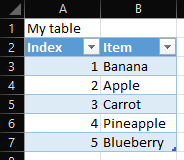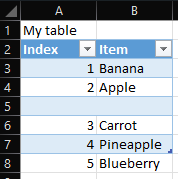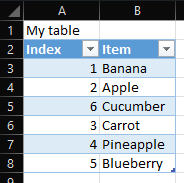# Inserting rows into Excel table without corrupting the table

## Inserting rows

With OpenPyXL you can insert rows into a worksheet with the worksheet method insert_rows. First, we need to load our table from the Excel workbook named "Table".

`from openpyxl import load_workbook`

`SAVE_FILENAME = "New Table.xlsx"`

`wb = load_workbook("Table.xlsx")`
`ws = wb.active`

Our Table workbook contains the following table starting from Cell A1 and the table size \$A\$2:\$B\$7:Now we are ready to insert our rows with insert_rows. insert_rows takes two arguments, `idx `and `amount`. The argument `idx `is the row number that you want to insert rows before. The `amount `gives the number of rows you want to insert. Let's insert one row before row 5.

`ws.insert_rows(idx=5, amount=1)`

Our table now looks like below, but wait! There is a problem. Our table does not include the last row anymore. The table size is still \$A\$2:\$B\$7. Remember that when you are inserting rows into an Excel workbook, Excel extends the table dimensions for you. OpenPyXL does not extend the table by default, so we need to change the tables reference.## Changing the table references

Ok, let's change the references. Our table name is Table1 in the Excel workbook. Changing the references is easy. While wer'e at it we can also insert new values in the table cells.

`table = ws.tables["Table1"]`
`table.ref = "A2:B8"`
`ws["A5"] = 6`
`ws["B5"] = "Cucumber"`

Much better! The source code is available on GitHub.